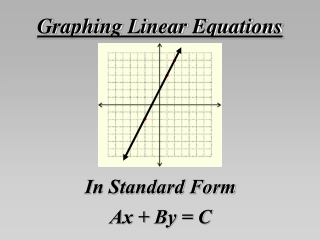DownloadDownload PresentationGraphing Linear Equations

# Graphing Linear Equations

Télécharger la présentation## Graphing Linear Equations

- - - - - - - - - - - - - - - - - - - - - - - - - - - E N D - - - - - - - - - - - - - - - - - - - - - - - - - - -
##### Presentation Transcript

1. Graphing Linear Equations In Standard Form Ax + By = C

2. Linear equations have two variables and when we plot all the (x,y) pairs that make the equation true we get a line. We will use The Standard Form of the equation to graph the line.

3. The formula for Standard Form is: Ax + By = C We can find 3 things just by looking at the formula: To find where the line crosses each axis, let the other value be zero. • If y is zero, we have Ax = C • is the x-intercept. • If x is zero, we have By = C • is the y-intercept. • − — is the slope. A B Using the x-intercept and/or y-intercept as a starting point, you can use the slope to graph more points on the line of the equation.

4. These equations are all in Standard Form: 2x – y = -1 x + y = -4 3x – 2y = 4 Notice that these equations are all in the form Ax + By = C.

5. right 1 up 2 right 1 up 2 2x – y = -1 • Find the x-intercept: • -1 ÷ 2 = -1/2 • 2) Find the y-intercept: • -1 ÷ -1 = 1 • 3) Plot the y-intercept. 4) Find the slope: -A/B − 2/-1 = 2 5) Plot more points using the slope.

6. up 1 down 1 right 1 left 1 x + y = -4 • Find the x-intercept: • -4 ÷ 1 = -4 • 2) Find the y-intercept: • -4 ÷ 1 = -4 • 3) Plot the x and y-intercepts. 4) Find the slope: -a/b − 1/1 = -1 5) Plot more points using the slope.

7. A graph with only one letter (x or y) only crosses that axis at that point. y = 5 ONLY crosses the y-axis… at 5 x = -3 ONLY crosses the x-axis… at -3

8. SUMMARY The formula for Standard Form is: Ax + By = C • If y=0,C ÷ A gives the • x-intercept • If x=0, C ÷ B gives the • y-intercept • − — is the • slope A B Using the x-intercept and/or y-intercept as a starting point, you can use the slope to graph the line of the equation.